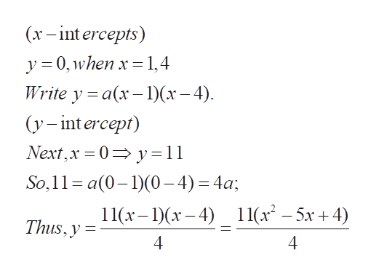# Find a formula for the quadratic function whose graph has a y-intercept of  y=11 and x-intercepts at  x=1,4.

Question
53 views

Find a formula for the quadratic function whose graph has a y-intercept of  y=11 and x-intercepts at  x=1,4.

check_circle

Step 1

To find the expression (second degree in x) for y from the given data

Step 2help_outlineImage Transcriptionclose(x-int ercepts) y0, when x 14 Write y a(x-1x-4) (y-intercept) Next,x 0 y11 So, 11 a(0-)(0-4) 4a Thus, y I(x-1)(x-4) _ 11(x2 - 5x+ 4) 4 4 fullscreen

### Want to see the full answer?

See Solution

#### Want to see this answer and more?

Solutions are written by subject experts who are available 24/7. Questions are typically answered within 1 hour.*

See Solution
*Response times may vary by subject and question.
Tagged in

### Equations and In-equations